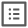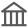××# 统计学理学硕士

Master of Science in Statistics综合排名： 23所属院校： 南加州大学

## 课程设置

1 数理统计学导论 Introduction to Mathematical Statistics
2 统计咨询研讨会 Seminar in Statistical Consulting
3 应用概率论 Applied Probability
4 方差分析与设计 Analysis of Variance and Design
5 时间序列导论 Introduction to Time Series
6 数值分析与计算 Numerical Analysis and Computation
7 数值分析 Numerical Analysis
8 数据分析 Data Analysis
9 概率论 Theory of Probability

## 预约咨询